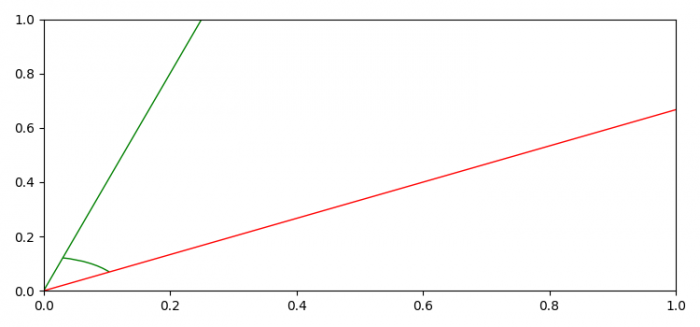# Best way to plot an angle between two lines in Matplotlib

The best way to plot an angle between two lines in Matplotlib is to use the Arc class to make an angle arc to plot the angle in between.

## Steps

• Set the figure size and adjust the padding between and around the subplots.
• Create a new figure or activate an existing figure using figure() method.
• Add an '~.axes.Axes' to the figure as part of a subplot arrangement using add_subplot() method.
• Create 2D line instances as l1 and l2.
• Add lines to the current axes.
• To plot an angle, call a user-defined method that returns an elliptical arc. Arc length could be created using slopes of the lines..
• To display the figure, use show() method.

## Example

from matplotlib import pyplot as plt, patches
import math

plt.rcParams["figure.figsize"] = [7.50, 3.50]
plt.rcParams["figure.autolayout"] = True

def angle_plot(line1, line2, offset=1.0, color=None, origin=(0, 0),
len_x_axis=1, len_y_axis=1):
xy1 = line1.get_xydata()
xy2 = line2.get_xydata()
slope1 = (xy1 - xy1) / float(xy1 - xy1)
angle1 = abs(math.degrees(math.atan(slope1)))
slope2 = (xy2 - xy2) / float(xy2 - xy2)
angle2 = abs(math.degrees(math.atan(slope2)))
theta1 = min(angle1, angle2)
theta2 = max(angle1, angle2)
angle = theta2 - theta1
if color is None:
color = line1.get_color()

return patches.Arc(origin, len_x_axis * offset, len_y_axis * offset, 0, theta1, theta2, color=color, label=str(angle) + u"\u00b0")

fig = plt.figure()

l1 = plt.Line2D([0, 1], [0, 4], linewidth=1, linestyle="-", color="green")
l2 = plt.Line2D([0, 4.5], [0, 3], linewidth=1, linestyle="-", color="red")

angle = angle_plot(l1, l2, 0.25)

plt.show()

## OutputUpdated on: 03-Jun-2021

865 Views